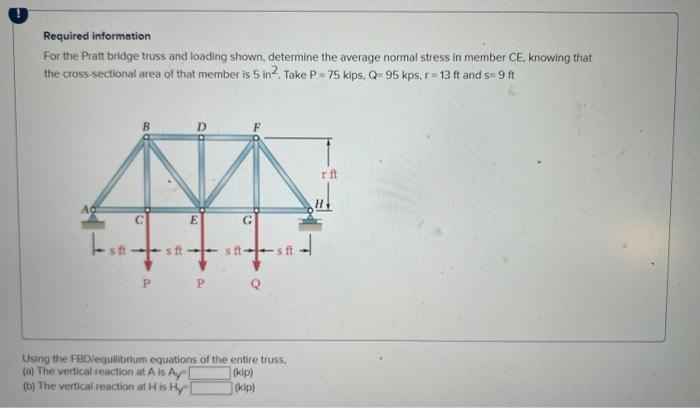# (Solved): Required informotion For the Pratt bridge truss and loading shown, determine the average normal str ...Required informotion For the Pratt bridge truss and loading shown, determine the average normal stress in member CE, knowing that the cross-sectional area of that member is 5 in 2 . Take $$P=75$$ kips, $$Q=95 \mathrm{kps}, \mathrm{r}=13 \mathrm{ft}$$ and $$\mathrm{s}=9 \mathrm{ft}$$ Using the FBDiequilibrium equations of the entire truss, (a) The vertical reaction at $$\mathrm{A}$$ is $$\mathrm{A}_{\mathrm{y}}$$ " (b) The verticai reaction at H is Hym (kip)

We have an Answer from Expert